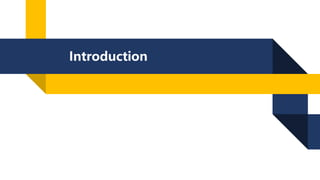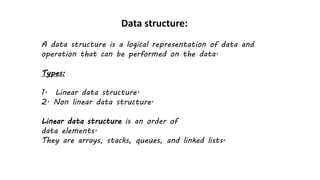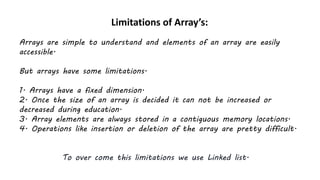Diese Präsentation wurde erfolgreich gemeldet.

# Different types of Linked list.

Anzeige
Anzeige
Anzeige
Anzeige
Anzeige
Anzeige
Anzeige
Anzeige
Anzeige
Anzeige
Anzeige×

1 von 15 Anzeige

# Different types of Linked list.

A linked list is a linear data structure where each element (node) is a separate object, connected to the previous and next elements by references. The first element is referred to as the head of the linked list and the last element is referred to as the tail. The nodes in a linked list can store data or simply act as a reference to the next node. Linked lists have several advantages, such as dynamic sizing and easy insertion and deletion of elements. They are commonly used in a variety of applications, such as implementing stacks, queues, and dynamic memory allocation.

There are several types of linked lists, including:

1. Singly linked list: Each node has a reference to the next node in the list.

2. Doubly linked list: Each node has a reference to both the next and previous node in the list.

3. Circular linked list: The last node in the list points back to the first node, creating a loop.

5. Doubly Circular linked list: Both the first and last node points to each other, forming a circular loop.

6. Skip list: A probabilistic data structure where each node has multiple references to other nodes.

7. XOR linked list: Each node stores the XOR of the addresses of the previous and next nodes, rather than actual addresses.

A linked list is a linear data structure where each element (node) is a separate object, connected to the previous and next elements by references. The first element is referred to as the head of the linked list and the last element is referred to as the tail. The nodes in a linked list can store data or simply act as a reference to the next node. Linked lists have several advantages, such as dynamic sizing and easy insertion and deletion of elements. They are commonly used in a variety of applications, such as implementing stacks, queues, and dynamic memory allocation.

There are several types of linked lists, including:

1. Singly linked list: Each node has a reference to the next node in the list.

2. Doubly linked list: Each node has a reference to both the next and previous node in the list.

3. Circular linked list: The last node in the list points back to the first node, creating a loop.

5. Doubly Circular linked list: Both the first and last node points to each other, forming a circular loop.

6. Skip list: A probabilistic data structure where each node has multiple references to other nodes.

7. XOR linked list: Each node stores the XOR of the addresses of the previous and next nodes, rather than actual addresses.

Anzeige
Anzeige

### Different types of Linked list.

1. 1. Introduction
2. 2. Data structure: A data structure is a logical representation of data and operation that can be performed on the data. Types: 1. Linear data structure. 2. Non linear data structure. Linear data structure is an order of data elements. They are arrays, stacks, queues, and linked lists.
3. 3. Limitations of Array’s: Arrays are simple to understand and elements of an array are easily accessible. But arrays have some limitations. 1. Arrays have a fixed dimension. 2. Once the size of an array is decided it can not be increased or decreased during education. 3. Array elements are always stored in a contiguous memory locations. 4. Operations like insertion or deletion of the array are pretty difficult. To over come this limitations we use Linked list.
4. 4. What is Linked list? Linked list is a linear data structure where elements are not stored sequentially inside the computer memory but they are linked with each other by the help of address.
5. 5. Linked list: Linked list is a collection of elements called nodes. Each node contains two parts. they are data part and link part. Data Link Node:
6. 6. Different types of Linked list -
7. 7. Singly Linked List: It is the simplest type of linked list in which every node contains some data and a pointer to the next node of the same data type. A single linked list allows the traversal of data only in one way.
8. 8. Algorithm: 1. Create a temporary node(temp) and assign the head node's address. 2. Print the data which present in the temp node. 3. After printing the data, move the temp pointer to the next node. 4. Do the above process until we reach the end.
9. 9. Operations: 1. Traversal. 2. Insertion. 3. Deletion. 4. Searching.
10. 10. Doubly Linked List: A doubly linked list is a bi-directional linked list. So, you can traverse it in both directions. Unlike singly linked lists, its nodes contain one extra pointer called the previous pointer. This pointer points to the previous node.
11. 11. Circular Linked List: While traversing a circular linked list, we can begin at any node and traverse the list in any direction forward and backward until we reach the same node we started. Thus, a circular linked list has no beginning and no end.
12. 12. Doubly Circular linked list: A circular doubly linked list is a mixture of a doubly linked list and a circular linked list. Like the doubly linked list, it has an extra pointer called the previous pointer, and similar to the circular linked list, its last node points at the head node. This type of linked list is the bi-directional list. So, you can traverse it in both directions.
13. 13. THANK YOU